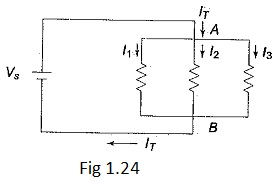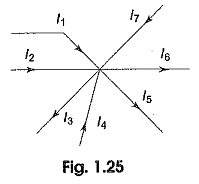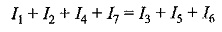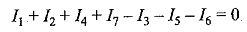# Kirchhoff’s Current Law

## Kirchhoff’s Current Law:

Kirchhoff’s Current Law states that the sum of the currents entering into any node is equal to the sum of the currents leaving that node. The node may be an interconnection of two or more branches. In any parallel circuit, the node is a junction point of two or more branches.The total current entering into a node is equal to the current leaving that node. For example, consider the circuit shown in Fig. 1.24, which contains two nodes A and B. The total current IT entering node A is divided into I1, I2, and I3.. These currents flow out of node A. According to Kirchhoff’s current law, the current into node A is equal to the total current out of node A: that is, IT=I1+I2+I3.. If we consider node B, all three currents I1, I2, and I3 are entering B, and the total current IT is leaving node B, Kirchhoff s current law formula at this node is therefore the same as at node A.In general, sum of the currents entering any point or node or junction equal to sum of the currents leaving from that point or node or junction as shown in Fig. 1.25.If all of the terms on the right side are brought over to the left side, their signs change to negative and a zero is left on the right side, i.e.This means that the algebraic sum of all the currents meeting at a junction is equal to zero.

Updated: September 30, 2017 — 2:28 pm

`Please enable JavaScript in your browser to complete this form.Name *FirstLastEmail *Paragraph TextMessage`
` `